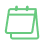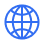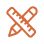### Functional skill Math Bundle

Functional Skills Math-A self-paced high-quality study Guide- Complete preparation with Worksheets, resources & Quizzes

Language: English

# About the package

Description

This bundle includes

1) Functional Skills Maths Level 1

2) Functional Skills Math Level 2

This Bundle is carefully designed to prepare students for the ‘Functional Skills Maths Level 1 & 2’. It will equip you with the desired knowledge and Math skills you will need in life, study and work.

Who is this Bundle for?

-Anyone interested in revising or learning the basics of Mathematics.

-Anyone who wants to excel at Functional Skills Math Level 1& 2 Exam

-People looking for an alternative GCSE Math qualification.

-Anyone looking to improve their job prospects by improving Math Skills.

Requirements

-Basic Addition, Subtraction, Multiplication, and Division

What are you going to learn?

-Number System, Negative Numbers, rounding off Numbers, Fractions, decimals, substitution in given Formula, money, Percentages, Simple interest, Length Mass & Capacity, TIME, Lines & Angles, Triangles & 2 d Figures, Line symmetry, work out the perimeter, area, and volume

-Ratio & Proportion, profit & Loss, Discounts & Taxes,  Compound Interest, Metric and Imperial units, compound Measures like Speed, Distance & Time, Density, Mass & Volumes, Rates of pay

-Prime factorization, HCF & LCM, ratios & direct proportions, Learn 1 step & 2 step formulae, Estimation, PEMDAS, Grouping of a discrete data, draw and interpret Bar Charts, Pie Charts, Finding Mean & Range, Probability on a scale from 0 to 1

-Perimeters, Areas of 2-D shapes, volumes & surface area of cubes and cuboids, Scale Drawings of actual measurements and scale factors, management of time, Problems based on money & currency, Statistics, Mean, Median, Mode & Range, Two-way Tables, Scatter Diagram and correlation, Probability of combined events

# What you’ll learn-Number System, Negative Numbers, rounding off Numbers, Fractions, decimals, substitution in given Formula, money, Percentages, Simple interest, Length Mass & Capacity, TIME, Lines & Angles, Triangles & 2 d Figures, Line symmetry, work out the perimeter, area, and volume-Ratio & Proportion, profit & Loss, Discounts & Taxes, Compound Interest, Metric and Imperial units, compound Measures like Speed, Distance & Time, Density, Mass & Volumes, Rates of pay-Prime factorization, HCF & LCM, ratios & direct proportions, Learn 1 step & 2 step formulae, Estimation, PEMDAS, Grouping of discrete data, draw and interpret Bar Charts, Pie Charts, Finding Mean & Range, Probability on a scale from 0 to 1-Perimeters, Areas of 2-D shapes, volumes & surface area of cubes and cuboids, Scale Drawings of actual measurements and scale factors, management of time, Problems based on money & currency, Statistics, Mean, Median, Mode & Range, Two-way Tables, Scatter Diagram and correlation, Probability of combined events

# Reviews and TestimonialsLaunch your Graphy
100K+ creators trust Graphy to teach online